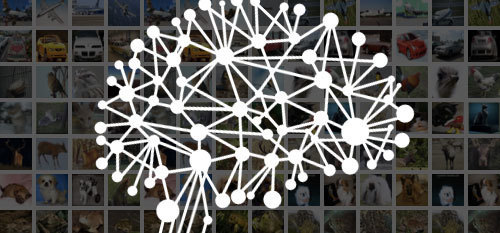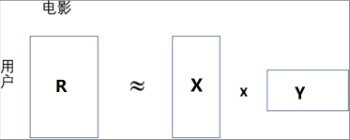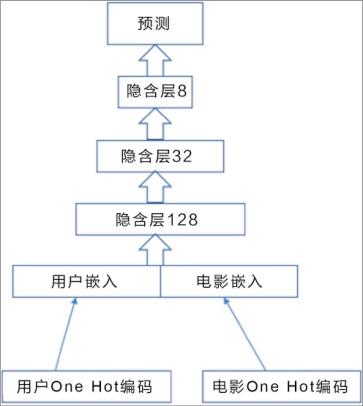# 推荐系统是如何建立模型、知道用户爱好的？

## 管理员账号

2017-08-08## 1.矩阵分解模型## 2.深度神经网络模型1 k = 128
2 model1 = Sequential()
3 model1.add(Embedding(n_users + 1, k, input_length = 1))
5 model2 = Sequential()
6 model2.add(Embedding(n_movies + 1, k, input_length = 1))

1 model = Sequential()
2 model.add(Merge([model1, model2], mode = ‘concat’))

model.compile(loss = ‘mse’, optimizer = “adam”)

1 users = ratings[‘user_id’].values
2 movies = ratings[‘movie_id’].values
5 推荐系统

label = ratings[‘rating’].values

1 X_train = [users, movies]
2 y_train = label

model.fit(X_train, y_train, batch_size = 100, epochs = 50)

1 i,j = 10,99
2 pred = model.predict([np.array([users[i]]), np.array([movies[j]])])

1 sum = 0
2 for i in range(ratings.shape):
3 sum += (ratings[‘rating’][i] - model.predict([np.array([ratings[‘user_id’
][i]]), np.array([ratings[‘movie_id’][i]])])) ** 2
4 mse = math.sqrt(sum/ratings.shape)
5 print(mse)

• ## #小编推书#唯一一本以应用为导向的介绍机器学习和深度学习的专业书籍

《Keras快速上手：基于Python的深度学习实战》从如何准备深度学习的环境开始，手把手地教读者如何采集数据，如何运用一些最常用，也是目前被认为最有效的一些深度学习算法来解决实际问题。覆盖的领域包括推荐系统、图像识别、自然语言情感分析...

878 0 1 0
• ## Keras 文字生成系统

同是深度学习“槛内人”，我怎么不知道这样高大上的文字生成对话系统 文字信息是存在最广泛的信息形式之一，而深度学习的序列模型（Sequential Model）在对文字生成建模（Generative Model）方面具备独特的优势。...

2116 0 0 0
• ## 推荐系统是如何建立模型、知道用户爱好的？

你是否有过这样的经历？当你在亚马逊商城浏览一些书籍，或者购买过一些书籍后，你的偏好就会被系统学到，系统会基于一些假设为你推荐相关书目。系统从百万甚至上亿的内容或商品中把有用的东西高效地显示给用户，这样可以为用户节省很多自行查询的时间，...

998 0 0 0